# Samacheer Kalvi 7th Maths Solutions Term 3 Chapter 2 Percentage and Simple Interest Ex 2.4

Students can Download Maths Chapter 2 Percentage and Simple Interest Ex 2.4 Questions and Answers, Notes Pdf, Samacheer Kalvi 7th Maths Book Solutions Guide Pdf helps you to revise the complete Tamilnadu State Board New Syllabus and score more marks in your examinations.

## Tamilnadu Samacheer Kalvi 7th Maths Solutions Term 3 Chapter 2 Percentage and Simple Interest Ex 2.4

Question 1.
Find the simple interest on ₹ 35,000 at 9% per annum for 2 years?
Solution: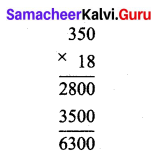Principal P = ₹ 35,000
Rate of interest r = 9 % Per annum
Time (n) = 2 years
Simple Interest I = $$\frac { Pnr }{ 100 }$$ = $$\frac{35000 \times 2 \times 9}{100}$$ = ₹ 6300
Simple intrest I = ₹ 6300Question 2.
Aravind borrowed a sum of ₹ 8,000 from Akash at 7% per annum. Find the interest and amount to be paid at the end of two years.
Solution:
Here Principal P = ₹ 8,000
Rate of interest r = 7% Per annum
Time (n) = 2 Years
Simple Interest (I) = $$\frac { Pnr }{ 100 }$$ = $$\frac{8000 \times 2 \times 7}{100}$$
I = ₹ 1120
Amount = P + I
I = ₹ 8000 + 1120 = 9120
Interest to be paid = ₹ 1,120
Amount to be paid = ₹ 9,120

Question 3.
Sheela has paid simple interest on a certain sum for 4 years at 9.5% per annum is ₹ 21,280. Find the sum.
Solution:
Let the Principal be ₹ P
Rate of interest r = 9.5% per annum
Time (n) = 4 years
Simple Interest I = $$\frac { Pnr }{ 100 }$$
Given I = ₹ 21,280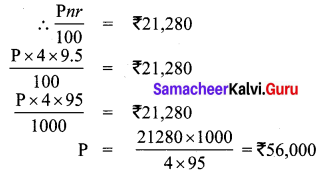∴ Sum of money Sheela bought = ₹ 56,000Question 4.
Basha borrowed ₹ 8,500 from a bank at a particular rate of simple interest. After 3 years, he paid ₹ 11,050 to settle his debt. At what rate of interest he borrowed the money?
Solution:
Let the rate of interest be r% per annum
Here Principal P = ₹ 8,500
Time n = 3 years
Total amount paid = ₹ 11,050
A = P + 1 = ₹ 11,050
i.e. 8,500 + 1 = ₹ 11,050
I = ₹ 11,050 – ₹ 8,500 = ₹ 2,550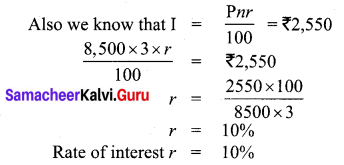Question 5.
In What time will ₹ 16,500 amount to ₹ 22,935 at 13% per annum?
Solution:
Rate of interest r = 13% per annum
Here Amount A = ₹ 22,935
Principal P = ₹ 16,500
A = P + I
22935 = 16,500 + I
∴ Interest I = 22935 – 16,500 = ₹ 6,435
Simple Interest I = $$\frac { pnr }{ 100 }$$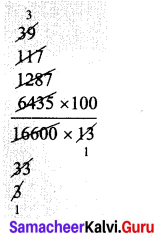6435 = $$\frac{16500 \times n \times 13}{100}$$
n =$$\frac{6435 \times 100}{16500 \times 13}$$
n = 3 years
Required time n = 3 years

Question 6.
In what time will ₹ 17800 amount to ₹ 19936 at 6% per annum?
Solution:
Let the require time be n years
Here Principal P = ₹ 17,800
Rate of interest r = 6% per annum
Amount A = ₹ 19,936
A = P + I
19936 = 17800 + 1
19936 – 17800 = I
2136 = I
Simple Interest (I) = $$\frac { pnr }{ 100 }$$
2136 = $$\frac{17800 \times n \times 6}{100}$$
n = $$\frac{2136 \times 100}{17800 \times 6}$$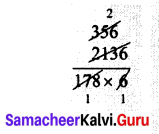n = 2 Years
Required time = 2 yearsQuestion 7.
A sum of ₹ 48,000 was lent out at simple interest and at the end of 2 years and 3 months the total amount was ₹ 55,560. Find the rate of interest per year.
Solution:
Given Principal P = ₹ 48,000
Time n = 2 years 3 months
= 2 + $$\frac { 3 }{ 12 }$$ years = 2 + $$\frac { 1 }{ 4 }$$ years
= $$\frac { 8 }{ 4 }$$ + $$\frac { 1 }{ 4 }$$ years = $$\frac { 9 }{ 4 }$$ years
Amount A = ₹ 55,660
A = p + 1
55660 = 48000 + I
I = 55660 – 48000 = ₹ 7660
∴ Interest for $$\frac { 9 }{ 4 }$$ years = ₹ 7660
Simple intrest = $$\frac { pnr }{ 100 }$$
7660 = 48000 × $$\frac { 9 }{ 4 }$$ × $$\frac { r }{ 100 }$$
r = $$\frac{7660 \times 4 \times 100}{9 \times 48000}$$ = 7.09 % = 7 %
Rate of interest = 7 % Per annum

Question 8.
A principal becomes ₹ 17,000 at the rate of 12% in 3 years. Find the principal.
Solution:
Given the Principal becomes ₹ 17,000
Let the principle initially be P
Rate of Interest r Time = 12 % Per annum
Time n = 3 years
According to the problem given I = 17000 – P = $$\frac{P \times 3 \times 12}{100}$$
17000 = $$\frac { 36 }{ 100 }$$ p + p
17000 = p($$\frac { 36 }{ 100 }$$ + 1)
17000 = p($$\frac { 136 }{ 100 }$$)
p = $$\frac{17000 \times 100}{136}$$ = 12,500
∴ Principal P = ₹ 12,500Objective Type Questions

Question 9.
The interest for a principle of? 4,500 which gives an amount of? 5,000 at end of certain period is
(i) ₹ 500
(ii) ₹ 200
(iii) 20%
(iv) 15%
Hint: Interest = Amount – Principle = ₹ 5000 – ₹ 4500 = ₹ 500
(i) ₹ 500

Question 10.
Which among the following is the simple interest for the principle of ₹ 1,000 for one year at the rate of 10% interest per annum?
(i) ₹ 200
(ii) ₹ 10
(iii) ₹ 100
(iv) ₹ 1,000
Hint: Intrest = $$\frac { pnr }{ 100 }$$ = $$\frac{1000 \times 1 \times 10}{100}$$ = ₹ 100Hint: r = $$\frac{I \times 100}{P \times n}$$ = $$\frac{200 \times 100}{2000 \times 1}$$ = 10 %